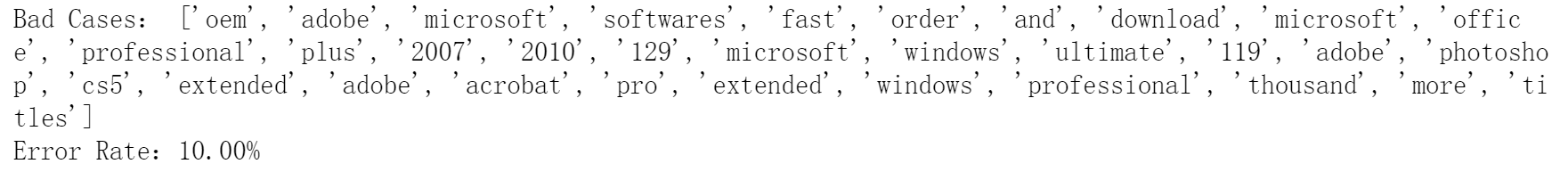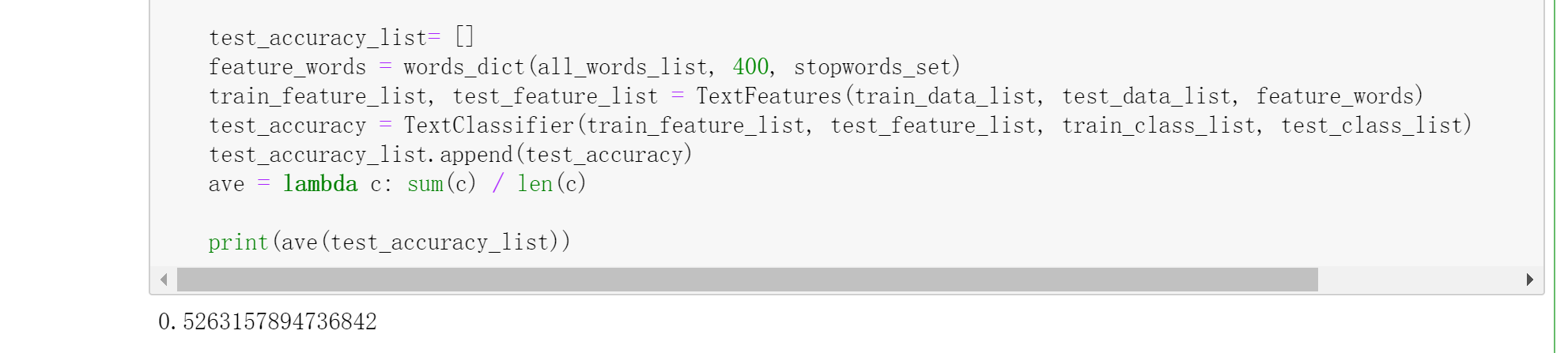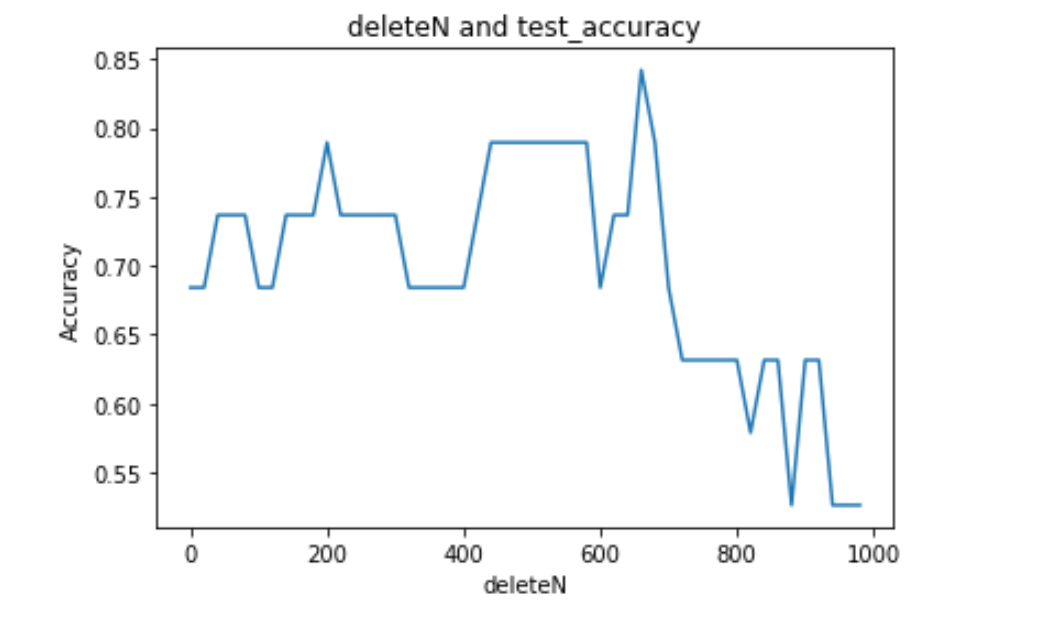# Halo

A magic place for coding

0%

## Intro

贝叶斯分类器是机器学习算法中非常重要的一个算法，它是基于朴素贝叶斯理论的。这篇博客中我将详细介绍贝叶斯分类器的由来，以及应用贝叶斯分类器做一个 [垃圾邮件过滤](# 垃圾邮件过滤) 和 [新闻分类](# 新闻分类)。

## 原理

### 基本思想

贝叶斯分类器对于分类问题一个最基本的思想是：** 谁的概率大就选谁 **。用数学语言描述，对于数据 $(x,y)$，类别 $P_1,P_2$：
$$\begin {cases} P_1 (x, y) \gt P_2 (x,y), 该数据为类别 1 \ P_1 (x,y) \lt P_2 (x, y), 该数据为类别 2 \end {cases}$$
在了解贝叶斯分类器之前，我们先来了解几个关于概率的基本概念。

### 条件概率

大家对于条件概率应该非常熟悉，这里就直接给出例子说明。
$$P (A|B) = \frac {P (A\cap B)}{P (B)}$$

我们根据上式进行推导：
$$\because P (B|A) = \frac {P (A\cap B)}{P (A)} \ \therefore P (A\cap B) = P (A|B) P (B)=P (B|A) P (A) \therefore P (A|B) = \frac {P (B|A) P (A)}{P (B)}$$
这样我们借助了 $P (A\cap B)$ 来建立了 $P (A|B)$ 和 $P (B|A)$ 之间的关系。

### 全概率公式

首先给出一条容易理解的等式：
$$P (B) = P (B\cap A) + P (B \cap A^{‘})$$

然后我们用 $P (B\cap A) = P (B|A) P (A)$ 替换，得到：
$$P (B) = P (B|A) P (A) + P (B|A^{‘}) P (A^{‘})$$

如果我们用全概率公式替换掉条件概率公式的分母，可以得到：
$$P (A|B) = \frac {P (B|A) P (A)}{P (B|A) P (A) + P (B|A^{‘}) P (A^{‘})}$$

### 贝叶斯推断

我们重新来看条件概率的公式，稍微调整一下分子的顺序：
$$P (A|B) = P (A)\cdot\frac {P (B|A)}{P (B)}$$
这样看的话，公式就可以分成三部分：

• $P (A)$：** 先验概率（prior probability）**，在事件 $B$ 发生前，我们对事件 $A$ 概率的一个判断。
• $P (A|B)$：** 后验概率（posterior probability）**，在事件 $B$ 发生后，我们对事件 $A$ 概率的重新评估。
• $\frac {P (B|A)}{P (B)}$：** 可能性函数（likelihood）**，一个调整因子，使得预估概率更接近真实的概率。我们来看看这个可能性函数的作用：
• $\frac {P (B|A)}{P (B)} \lt 1$：先验概率被削弱，事件 $A$ 可能性变小；
• $\frac {P (B|A)}{P (B)} = 1$：事件 $B$ 无助于判断事件 $A$ 的可能性；
• $\frac {P (B|A)}{P (B)} \gt 1$：先验概率被增强，事件 $A$ 可能性变大。

我们用一个例子来说明这些概念的作用。假设现在有两个一模一样的箱子，第一个箱子中有 30 个黑球，10 个白球；第二个箱子中有 20 个黑球，20 个黑球。请问，从两个箱子中摸出了一个球，确定是黑球，那么这个黑球来自第一个箱子的概率有多大？

我们定义 $P (H_1),P (H_2)$ 分别为从第一个箱子和第二个箱子取出球的概率。可以知道，$P (H_1)=P (H_2)=0.5$。这个就是 ** 先验概率 **。然后我们定义 $P (E)$ 为取出黑色球的概率。这样就能够根据上面写的公式来表示我们要求的概率了：
$$P (H_1|E)=P (H_1)\frac {P (E|H_1)}{P (E)} = \frac {P (H_1)\cdot P (E|H_1)}{P (E|H_1) P (H_1) + P (E|H_2) P (H_2)} = \frac {0.5 \times 0.75}{0.75 \times 0.5 + 0.5 \times 0.5} = 0.6$$
也就是说，取出黑球这个事件增强了从第一个箱子取出黑球的可能性，即先验概率被增强了。

### 朴素贝叶斯

朴素贝叶斯和上面的概念是完全不一样的，它的主要内容是：对条件各概率分布做了条件独立性的假设，即条件之间是相互独立的。假设有 $n$ 个特征，那么有：
\begin {aligned} P (a|X) &= P (X|a) P (a)=P (x_1, x_2,x_3,\cdots,x_n|a) P (a) \ &= {p (x_1|a) \times p (x_2|a) \times p (x_3|a) \times \cdots \times p (x_n|a)} p (a) \end {aligned}
把各个条件独立分开对于计算是非常有好处的，这也是贝叶斯分类器可以实现的统计学基础。

### 贝叶斯分类器

基于朴素贝叶斯的思想，贝叶斯分类器的基本方法是：在统计资料的基础上，依据某些特征，计算各个类别的概率，从而实现分类。

## 实战

### 垃圾邮件过滤

垃圾邮件过滤是机器学习的经典应用场景。这里利用贝叶斯分类器对邮件进行过滤。数据源：https://github.com/leungyukshing/Machine-Learning/tree/master/Bayes/email

#### 邮件文本预处理

我们需要对邮件文本进行预处理，将文本切分成单词，然后使用所有的单词去构建起一个 ** 词典 **。我们的目的是根据单词去判断一个邮件是否垃圾邮件。

#### 文本向量化

我们拥有了词典，但是在计算概率的过程中使用字符串还是比较复杂，因此我们需要将文本向量化 —— 即用一个向量来表示一个文本，向量每个元素对应字典里面的每个单词，如果这个单词在该文本中出现了，那么在向量的对应位置上就为 1，否则为 0。

#### 计算概率

现在我们来看看整个分类问题的核心是什么：
$$P (类别 |{单词 1，单词 2 \cdots ，单词 n}) = {P (单词 1 | 类别) \times P (单词 2 | 类别) \times P (单词 3 | 类别) \times \cdots \times P (单词 n | 类别)} P (类别)$$

先看代码，然后再逐步分析：

这里就是计算上述公式中右边部分的代码。p1Num 统计的是在已知垃圾邮件的情况下，各单词的出现次数，是一个向量。p1Denom 统计的是所有垃圾邮件单词的总数量，两个的比值是一个向量，里面存放着就是垃圾邮件的条件概率，即 $P (每个单词 | 垃圾邮件)$。

为什么计算条件概率的时候初始化不是初始化为 0 呢？我们还是看回公式：
$$P (类别 |{单词 1，单词 2，\cdots ，单词 n}) = {P (单词 1 | 类别) \times P (单词 2 | 类别) \times P (单词 3 | 类别) \times \cdots \times P (单词 n | 类别)} P (类别)$$
如果某一个单词的条件概率为 0，即 $P (单词 x | 类别) = 0$，那么就会导致整条式子为 0。也就是说，在实际应用中，如果有些单词的条件概率为 0 或者很小，我们这个方法就行不通了。

于是我们进行微处理 ——** 拉普拉斯平滑（Laplace Smoothing）**。它是比较常见的平滑方法，做法就是将分子初始化为 1，分母初始化为 2。

上面提到实际应用中，精度问题也是必须考虑的问题之一，如果这些条件概率很小，那么相乘之后就会有精度问题。这里的处理是用一个对数的方法，避免精度产生的问题。

#### 测试

我们将所有的数据（共 50 份）分为两部分，40 个训练集，10 个测试集。然后使用训练集构建贝叶斯分类器，对 10 个测试样例进行测试，最后得到结果。

#### 结果

最终的测试结果是比较好的，基本都在 10% 以内，大多数情况下都能完全分类正确。### 新闻分类

除了上面应用在垃圾邮件分类上，贝叶斯分类器还能用在新闻分类中。原理是很相似的，也是根据词语的不同来计算条件概率。这里我们尝试对中文文本进行分类，而且类别也相应地增加。

#### 新闻文本预处理

我们处理的是中文文本，所以需要先对文本进行读取和切分，这里用到了一个中文分词的库 ——jieba。类似地，我们也是通过构建词典来将文本向量化。

这里数据集已经给出了常见的结束词语，我们需要在字典中去除掉这些词语，首先构建出结束词语的词典。

#### 精简词典

上面的步骤就和垃圾邮件过滤一样，提取出来了所有的词语，构成了一个词典。但是这个词典的好坏对于结果的影响是非常重大的。我们可以 print 一下，发现这个词典中大量的高频词都是一些无实际意义的词语，如 “在”、“了”、” 很 “。这些助词、介词对于文本的分类并没有实质的作用，因此我们需要把这些无意义的高频词去除掉，否则将严重影响分类结果。

处理的原则有三个：

• 去除数字
• 去除在结束词语列表中的词语
• 去除长度过短或过长的词语

#### 文本向量化

这里的文本向量化思想与垃圾邮件分类是一样的。

#### 构建贝叶斯分类器

上面我们自己实现了贝叶斯分类器，这里我们试一下使用 sklearn 提供的贝叶斯分类器。

#### 测试及优化

我们可以运行测试，看到结果：注意我之前提到过，词典的质量影响着分类的准确度，这里删掉前 400 个高频词（是随便调的一个参数），那么比较好的是 deleteN 是多少呢？我们可以自己写一个循环去找到这个最优参数。

我从 0 一直到 1000，以步长为 20 去搜索这个最优的 deleteN，输出 deleteN-Accuracy 曲线：从图中可以大致看出，最优的 deleteN 应该是在 600-700 之间。所以调整参数为 680，再次测试：

## 总结

至此，我就把贝叶斯分类器的一些基础知识分享给大家了，借用了两个实例来应用了一下贝叶斯分类器（既有自己实现的，也有调用 sklearn 的）。相信通过这篇博客，大家贝叶斯分类器有了一定了解。欢迎提出宝贵的意见，谢谢您的支持！

Welcome to my other publishing channels